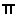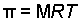Calculating Osmotic Pressure

Osmotic Pressure

We need to know the molar concentration of dissolved species in order to calculate the osmotic pressure of an aqueous solution. We calculate the osmotic pressure,(pi), using the following equation:Where:

M is the molar concentration of dissolved species (units of mol/L).
R is the ideal gas constant (0.08206 L atm mol-1 K-1, or other values depending on the pressure units).
T is the temperature on the Kelvin scale.